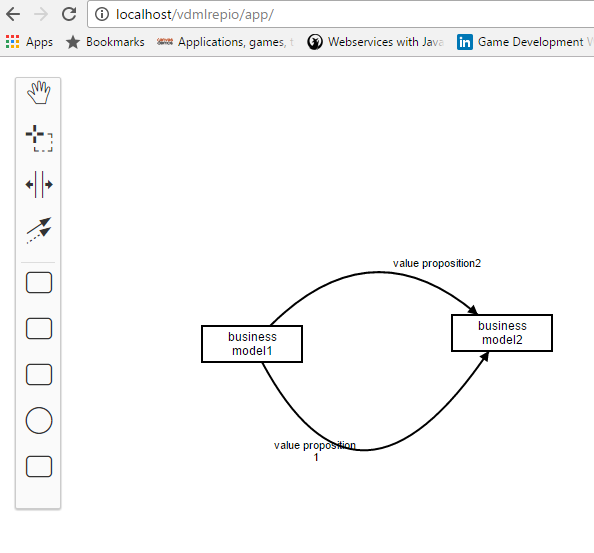# Bezier Curve drawing

Has any one tried drawing bezier curve using diagramjs? is there api/way to do it already?

Thanks and Regards
Rajender

Hello @Rajender,

we haven’t got any API related to drawing curves.

Cheers,
Ricardo

ok THanks… I got this working.

Could you provide an example for others interested how you got things working?

yes, sure…will post it this week

``````function toSVGPoints(points) {
var result = '';

for (var i = 0, p; (p = points[i]); i++) {
result += p.x + ',' + p.y + ' ';
}

return result;
}

function toSVGCurve(points) {
var result = '';
var result = 'm  ' + points.x + ',' + points.y;
if (!isCurve) {
for (var i = 1; i < points.length; i++) {
result += 'L' + points[i].x + ',' + points[i].y + ' ';
}
} else {
if (points.length >= 3) {
result += ' Q' + points.x + ',' + points.y;
result += ' ' + points.x + ',' + points.y;
}
for (var i = 3, p; (p = points[i]) ; i++) {
result += ' T' + points[i].x + ',' + points[i].y;
}
}

return result;
}

module.exports.createCurve = function (points, attrs) {
return Snap.create('path', { d: toSVGCurve(points) }).attr(attrs || {});
};

module.exports.updateCurve = function (gfx, points) {
return gfx.attr({ d: toSVGCurve(points) });
};
In the RenderUtil.js of diagram.js

And in the modeler renderer

function isCurvedConnection(connection) {
if (connection.type === 'vdml:SequenceFlow') {
return true;
}
return false;
}
function createPathFromConnection(connection) {
var isCurve = isCurvedConnection(connection);
var waypoints = connection.waypoints;

var pathData = 'm  ' + waypoints.x + ',' + waypoints.y;
if (!isCurve) {
for (var i = 1; i < waypoints.length; i++) {
pathData += 'L' + waypoints[i].x + ',' + waypoints[i].y + ' ';
}
} else {
if (waypoints.length >= 3) {
pathData += ' Q' + waypoints.x + ',' + waypoints.y;
pathData += ' ' + waypoints.x + ',' + waypoints.y;
}
for (var i = 3, p; (p = waypoints[i]) ; i++) {
pathData += ' T' + waypoints[i].x + ',' + waypoints[i].y;
}
}
return pathData;
}
``````

Used the above code to draw curve…

2 Likes

Thanks for sharing your solution with us.

Hello @Rajender,

how does a process with bezier curve look like? I’m curious. Could you please post a picture?

Cheers, Ingo

Please find attached a screen shot of a new modeller I am working on…this shows the value propositions being exchanged between 2 business modelsMy variant of smooth connection rendering is based on this StackOverflow answer (http://stackoverflow.com/questions/7054272/how-to-draw-smooth-curve-through-n-points-using-javascript-html5-canvas).

``````    var waypoints = connection.waypoints;

var pathData = 'm  ' + waypoints.x + ',' + waypoints.y;
if (waypoints.length === 2) {
pathData += ' L' + waypoints.x + ',' + waypoints.y;
return pathData;
} else {
// http://stackoverflow.com/questions/7054272/how-to-draw-smooth-curve-through-n-points-using-javascript-html5-canvas
pathData += 'Q';
var i;
for (i = 1; i < waypoints.length - 2; i++) {
var xc = (waypoints[i].x + waypoints[i + 1].x) / 2;
var yc = (waypoints[i].y + waypoints[i + 1].y) / 2;
pathData += ' ' + waypoints[i].x + ',' + waypoints[i].y;
pathData += ' ' + xc + ',' + yc;
}
pathData += ' ' + waypoints[i].x + ',' + waypoints[i].y;
pathData += ' ' + waypoints[i+1].x + ',' + waypoints[i+1].y;
}
return pathData;
``````

Thank you…this looks smother.Only issue is we could also have no of waypoints >2 but still have straight lines…so we need another parameter to say if its a curve.

Hey, could you outline how you achieve this a little bit more? Where would I have to enter this to get started?
Thanks!